Engineering Mechanics - Force Vectors

Why Engineering Mechanics Force Vectors?

In this section you can learn and practice Engineering Mechanics Questions based on "Force Vectors" and improve your skills in order to face the interview, competitive examination and various entrance test (CAT, GATE, GRE, MAT, Bank Exam, Railway Exam etc.) with full confidence.

Where can I get Engineering Mechanics Force Vectors questions and answers with explanation?

IndiaBIX provides you lots of fully solved Engineering Mechanics (Force Vectors) questions and answers with Explanation. Solved examples with detailed answer description, explanation are given and it would be easy to understand. All students, freshers can download Engineering Mechanics Force Vectors quiz questions with answers as PDF files and eBooks.

Where can I get Engineering Mechanics Force Vectors Interview Questions and Answers (objective type, multiple choice)?

Here you can find objective type Engineering Mechanics Force Vectors questions and answers for interview and entrance examination. Multiple choice and true or false type questions are also provided.

How to solve Engineering Mechanics Force Vectors problems?

You can easily solve all kind of Engineering Mechanics questions based on Force Vectors by practicing the objective type exercises given below, also get shortcut methods to solve Engineering Mechanics Force Vectors problems.

Exercise :: Force Vectors - General Questions

• Force Vectors - General Questions
1.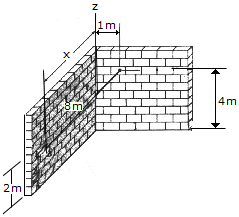The cord is attached between two walls. If it is 8 m long, determine the distance x to the point of attachment at B.

 A. x = 7.75 m B. x = 7.68 m C. x = 6.93 m D. x = 7.94 m

Explanation:

No answer description available for this question. Let us discuss.

2.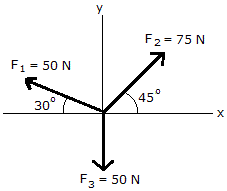Determine the magnitude of the resultant force by adding the rectangular components of the three forces.

 A. R = 29.7 N B. R = 54.2N C. R = 90.8 N D. R = 24.0 N

Explanation:

No answer description available for this question. Let us discuss.

3.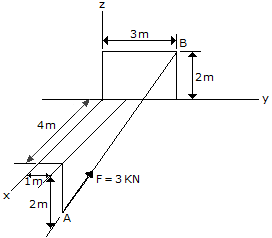Express force F as a Cartesian vector; then determine its direction angles.

 A. F = (-2i +j+2k) kN,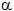= 48.2°,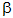= 70.5°,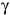= 48.2° B. F = (-2i +j+2k) kN,= 131.8°,= 70.5°,= 48.2° C. F = (-4i +j+4k) kN,= 48.2°,= 70.5°,= 48.2° D. F = (-4i +2j+4k) kN,= 131.8°,= 70.5°,= 48.2°

Explanation:

No answer description available for this question. Let us discuss.

4.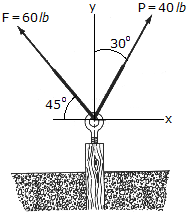Determine the magnitude and direction of the resultant force.

 A. R = 80.3 lb,= 106.2° CCW B. R = 80.3 lb,= 73.8° CCW C. R = 72.1 lb,= 63.6° CCW D. R = 72.1 lb,= 116.4° CCW

Explanation:

No answer description available for this question. Let us discuss.

5.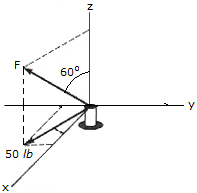Force F acts on peg A such that one of its components, lying in the x-y plane, has a magnitude of 50 lb. Express F as a Cartesian vector.

 A. F = (43.3 i + 25.0 j + 25.0 k) lb B. F = (43.3 i + 25.0 j + 28.9 k) lb C. F = (43.3 i - 25.0 j + 25.0 k) lb D. F = (43.3 i - 25.0 j + 28.9 k) lb